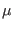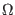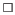Next: Default Models Up: WRspice Input Format Previous: Circuit Elements   Contents   Index

# Device Models

Many devices reference models, which contain values for the numerous parameters describing the device, which would be cumbersome to include in each device reference. Device models are specified on a .model line. The model can be referenced by any number of devices of the corresponding type.

General Form:
.model modname type (pname1=pval1 pname2=pval2 ... )
Examples:
.model mod1 npn (bf=50 is=1e-13 vbf=50)
.model intercon ltra (r=0.2 l=9.13nh c=3.65pf len=5 rel=.002 compactrel=1.0E-4)

The .model line specifies a set of model parameters that will be used by one or more devices. The modname is the model name, which is case insensitive in matching references, and type is one of the following types:

 c Capacitor model l Inductor model r Resistor model sw Voltage-controlled switch model csw Current-controlled switch model tra General transmission line model ltra Lossy transmission line model urc Uniform RC line model d Diode model npn NPN BJT model pnp PNP BJT model njf N-channel JFET model pjf P-channel JFET model nmf N-channel MESFET model pmf P-channel MESFET model nmos N-channel MOSFET model pmos P-channel MOSFET model jj Josephson junction model

Parameter values are defined by appending the parameter name, as given for each model type, followed by an equal sign and the parameter value. Model parameters that are not given a value are generally assigned default values.

The show command with the -M option is useful for listing the parameters that can be specified to a model. Only the parameters not listed as RO'' (read-only) can appear in a .model line.

In the tables that follow, the various model parameters are listed. The units'' field of the tables provides the assumed units of measure for the parameter, which is expressed using symbols from the following table.

 M meters cM centimetersM microns S seconds Hz hertz F farads H henriesohms C degrees Celsiussquare A amperes V volts eV electron-volts deg degrees

SubsectionsNext: Default Models Up: WRspice Input Format Previous: Circuit Elements   Contents   Index
Stephen R. Whiteley 2022-09-18